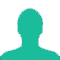# A person sold a horse at a gain of 15%. Had he bought it for 25% less and sold it for Rs. 600 less, he would have made a profit of 32%. The cost price of the horse was:

A. Rs. 3,750

B. Rs. 3,250

C. Rs. 2,750

D. Rs. 2,250

### Solution(By Examveda Team)

\eqalign{ & {\text{Let}}\,{\text{the}}\,{\text{original}}\,{\text{CP}} = {\text{Rs}}.\,X \cr & {\text{Hence}}, \cr & {\text{SP}} = X + 15\% \,{\text{of}}\,X \cr & \,\,\,\,\,\,\,\,\, = \frac{{115X}}{{100}} \cr & \,\,\,\,\,\,\,\,\, = Rs.\,\frac{{23x}}{{20}} \cr & {\text{New}}\,{\text{CP}} = x - 25\% \,{\text{of}}\,X \cr & = \frac{{75x}}{{100}} = \frac{{3x}}{4} \cr & {\text{New}}\,{\text{SP}} = \frac{{3x}}{4} + 32\% \,{\text{of}}\,\frac{{3x}}{4} \cr & = Rs.\,\frac{{99x}}{{100}} \cr & \cr & {\text{According}}\,{\text{to}}\,{\text{the}}\,{\text{question,}} \cr & {\frac{{23x}}{{20}}} - {\frac{{99x}}{{100}}} = 600 \cr & {\text{Or}},\,\frac{{ {115x - 99x} }}{{100}} = 600 \cr & 16x = 600 \times 100 \cr & X = 600 \times \frac{{100}}{{16}} \cr & \,\,\,\,\,\, = Rs.\,3750 \cr}

1.Cp(let)100__100+15____115%(sp1)
Sp 100-25=75
Profit %32 nikalna ha ok
75×32÷100=24
New sp2- 75+24=99
115-99=16p
16p-600
600÷16×100=3750

New

2.OLD. NEW
CP. 100. (100-25)=75
Profit 15. 75*32/100=24
SP. 115. 99
Difference between old and new SP, 115-99=16
Since, difference= 600
Therefore. 16=600
Now to calculate CP,
(16/100)=(600/CP)
CP= 3750

3.CP₁ : SP₁ = 20 : 23
CP₂ : SP₂ = 15 : 19.8
3.2 ≡ 20 ,Then 600 ≡ 3750

4.sp1 - sp2 = 600 ---------- (1)
now let, cp = x
sp1 = (115/100)x = 23x / 20
new cp = (75/100)x = 3x/4
so sp2 = (132/100)(3x/4) = 99x/100
Now put the value eq(1)
then x = 3750(ans)

5.let, cp = 100
sp = 115
new cp = 75
new sp = 75+ 75*(32/100)
= 99
sp difference = 115- 99 = 16
Atq, when diff. 16 then cp = 100
" " 1 " " = 100/16
" " 600 " " = (100*600)/16
= 3750

6.It's very lengthy... Give short cut.plz.

7.let CP=100x
so SP=115x.
now, according to question new CP=75x.
new SP=115x-600.
applying formula
115x-600=132 /100 X 75x
x=75/2.
CP=100 X 75/2=3750

8.1st condition
let he purchased it for 100. therefore his selling price is 115
2nd condition
if he bought it for 25% less, means => let he bought it for 75 then his gain is 32%of 75= 24. => his selling price is 99

C.P S.P
100 115
75 99

difference b/w S.P is 115-99= 16

c.p difference
100 16
? 600

cross multiply this and the answer is 3750.

9.plzz explain how this new sp came in step 4

Related Questions on Profit and Loss